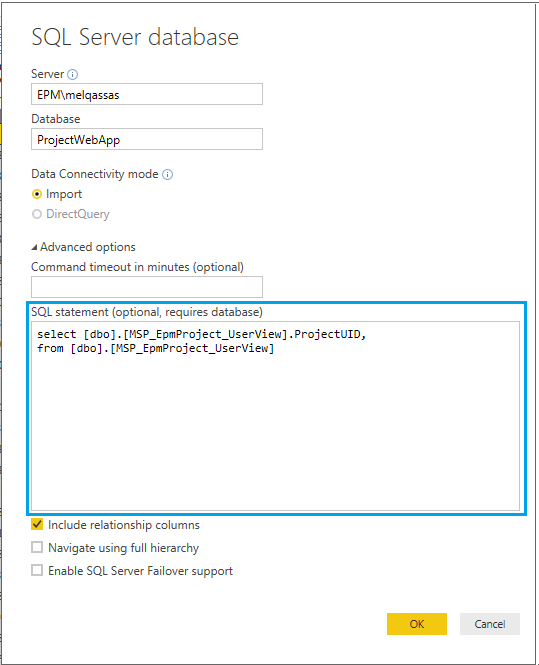# Convert this query to dax formulas

0 like 3 dislike
720 views
in Power BI 2 flags
reopened

I have the below complex SQL query and I need to convert this SQL query to DAX formulas in Power BI desktop, How I can do that?

```select

departmentName,

sum(ProductivityGain) ProductivityGainHours, sum(ProductivitySavings) ProductivitySavings,

sum(leavesGranted)  leavesGrantedDays,

sum(leavesTaken) leavesTakenHours,

round(100.00*(sum(leavesTaken) /  (sum(leavesGranted)*8)),3) leaveRate,

(sum(leavesGranted) - sum(leavesTaken)/8) /  sum(headCount) avgLeaveRemainingDaysPerEmployee,

round((avg(hourlyRate)*((sum(leavesGranted) - sum(leavesTaken)/8)) /  sum(headCount)),3) avgLeaveRemainingPotentialSavingsPerEmployee,

from

(select f.employeeid,f.GlobalclientId clientId, d.departmentName, l.leaveType,

case

when datepart(year,dateOfBirth) between 2013 and 2025 then 'Gen Alpha'

when  datepart(year,dateOfBirth) between 1995 and 2012 then 'Gen Z'

when  datepart(year,dateOfBirth) between 1980 and 1994 then 'Gen Y'

when  datepart(year,dateOfBirth) between 1966 and 1979 then 'Gen X'

when  datepart(year,dateOfBirth) between 1946 and 1965 then 'Baby Boomers'

when  datepart(year,dateOfBirth) between 1925 and 1945 then 'Silent Generation'

when  datepart(year,dateOfBirth) < 1925 then 'Greatest Generation'

when  datepart(year,dateOfBirth) > 2025 then 'To Be Determined'

end ageGeneration,

case

when year(getdate()) - year(dateOfBirth) between 13 and 18 then '13 - 18'

when  year(getdate()) - year(dateOfBirth) between 19 and 27 then  '19 - 27'

when  year(getdate()) - year(dateOfBirth) between 28 and 35 then  '28 - 35'

when  year(getdate()) - year(dateOfBirth) between 36 and 45 then  '36 - 45'

when  year(getdate()) - year(dateOfBirth) between 46 and 55 then  '46 - 55'

when  year(getdate()) - year(dateOfBirth) between 56 and 65 then  '56 - 65'

when  year(getdate()) - year(dateOfBirth) >= 66 then  '65+'

end ageGroup,

l.absentReason,

dates.[year] dimYear, dates.[month] dimMonth,

f.email, FirstDayOfMonth,

max(annualSalary) annualSalary ,

(sum(f.annualLeaveAllocated+MedicalLeaveAllocated+ParentLeaveAllocated+CasualLeaveAllocated+LongServiceLeaveAllocated)*8 -

isnull(sum(l.hours),0)) * sum(f.annualSalary/c.[AverageYearlyWorkingHours]) ProductivitySavings,

sum(f.annualLeaveAllocated+MedicalLeaveAllocated+ParentLeaveAllocated+CasualLeaveAllocated+LongServiceLeaveAllocated)*8 ProductivityGain,

--,

sum(f.annualSalary/c.[AverageYearlyWorkingHours]) hourlyRate,

--

cast(sum(f.annualLeaveAllocated+MedicalLeaveAllocated+ParentLeaveAllocated+CasualLeaveAllocated+LongServiceLeaveAllocated) as decimal(28,2)) leavesGranted,

cast(isnull(sum(l.hours),0) as decimal(28,2)) leavesTaken,

sum(f.annualLeaveAllocated) -

(sum(case

when leaveType = 'Annual Leave' then [hours]

else 0

end) / 8 ) annualLeaveRemaingDays,

sum(f.medicalLeaveAllocated) -

(sum(case

when leaveType = 'Medical Leave' then [hours]

else 0

end) / 8 ) medicalLeaveRemaingDays

--

from hr.factHr f

cross join

(select distinct [year], LastDayOfMonth, FirstDayOfMonth, [month] from shared.dateDimension) dates

left outer join hr.leaves l

on l.employeeId = f.employeeId

and [date]   <= LastDayOfMonth

cross join hr.companyDetail c

inner join hr.department d

on d.departmentId = f.departmentId

where

dateofJoining <= LastDayOfMonth

and

( terminationDate is null

or terminationDate > LastDayOfMonth)

group by f.Globalclientid ,d.departmentName,leaveType, dateOfBirth,

absentReason,

f.employeeid,f.email, dates.[year], dates.[month],FirstDayOfMonth) dataset

where dimYear = 2018

and dimMonth = 1

group by departmentName```

2 like 0 dislike
by 64 137 299

## Convert Complex SQL Query to DAX

As I mentioned at Convert SQL Query to DAX Formula in Power BI, DAX operates in a very different way from SQL Query! there is no direct way to convert such complex SQL queries to DAX.